# Vigenère Cipher – Finding the Key Length

No progress can be made in cracking a Vigenère cipher until the length of the key has been determined. We will now look at the second way of doing this.

#### Finding the key length by using the Index of Coincidence

The index of coincidence is a very useful mathematical tool to give you an early insight into the secrets of a coded message. Imagine all the letters from a cipher text have been copied onto Scrabble letter tiles, one tile for every letter in the message.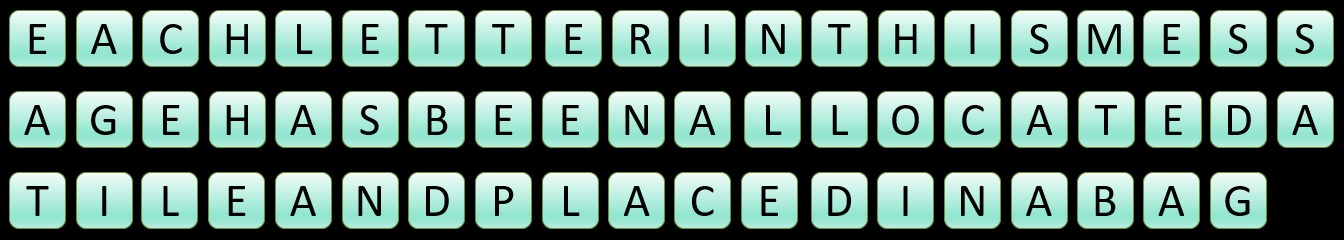These tiles are then placed in a bag and you are allowed to remove two tiles from it.The question that the index of coincidence is concerned with is this: what is the probability that both of these tiles show the same letter?

It is surprising that such an apparently dull question can prove so useful ... Let's see how.

#### The usefulness of the Index of Coincidence

Take a text that consists of only one letter:

AAAAAAAAAAAAAAAAAAAAAAAAAAAAAAAAAAAAAAAAAAAAAAAAAAAAAAAAAAAAAAAAAAAAAAAAAAAAAAAAAAAAAAAAAAAAAAAAAAAAAAAAAAAAAAAAAAAAAAAAAAAAAAAAAAAAAAAAAAAAAAAAAAAAAAAAAAAAAAAAAAAAAAAAAAAAAAAAAAAAAAA

If each of these 'A's was allocated a tile and we then randomly drew two tiles from the finished pile, then the probabilitiy that we will have two letters the same is 1.0, i.e. it is certain to happen. It does not matter which letter of the alphabet we use, we will always get an answer of 1.0.

Let's go to the other extreme, and pick a text where every letter occurs the same number of times:

ABCDEFGHIJKLMNOPQRSTUVWXYZABCDEFGHIJKLMNOPQRSTUVWXYZABCDEFGHIJKLMNOPQRSTUVWXYZABCDEFGHIJKLMNOPQRSTUVWXYZABCDEFGHIJKLMNOPQRSTUVWXYZABCDEFGHIJKLMNOPQRSTUVWXYZABCDEFGHIJKLMNOPQRSTUVWXYZ

Below, we will define such a text as 'random text'. For the moment, imagine that this random text keeps repeating the alphabet an infinite number of times (see below for more mathematical detail). If we draw just one letter from the bag, then each letter has an equal chance of being drawn from the bag, i.e. a 1 in 26 chance. If the text was infintely long, then the chance of drawing two letters that are the same is also 1 in 26, or approximately 0.0385 (again, see below for further explanation).

So we now have our two extremes:

• Index = 1.0000 - text with no randomness at all (i.e. all letters are the same)
• Index = 0.0385 - completely random text

What about typical English text? It obviously falls somewhere between these two values. It is not completely random because not all letters have an equal chance of appearing - there are far more E's than Z's, for example, in a typical passage of English (see here for more on letter frequencies in English). And it is also very different from a message consisting of just one letter. Providing the message is long enough for the typical features of the English language to manifest themselves, the index of coincidence for typical English comes out at about 0.0660. So now we have:

• Index = 1.0000 - text with no randomness at all (i.e. all letters are the same)
• Index = 0.0660 (approx.) - text written in English
• Index = 0.0385 - completely random text

We are now ready to appreciate the first way in which the index of coincidence is useful:

If a message has been encoded using a monoalphabetic cipher (see here for more information), such as a Caesar cipher or simple substitution cipher, then the index of coincidence remains unchanged. Or, if a message has been encoded with a type of transposition cipher, i.e. one which rearranges the letters of the original message than changing them, then the index of coincidence also remains unchanged.

What this means is that if you work out the index of coincidence for a cipher text and it comes out at around 0.0650 or higher, then there is a good chance that the message has been encoded using either a Caesar cipher, a simple substitution cipher or a transposition cipher.

This website automatically works out the index of coincidence for a message for you. Click here and copy a cipher text in the top box. Then, press TAB or click somewhere else on the screen. The index of coincidence for the message will be displayed near the top of the left side panel.

A simple transposition cipher simply rearranges the letters of a message, so, as the index of coincidence is worked out based on the frequencies of each letter in the message rather than their position, it is easy to see why the index of coincidence remains unchanged. Simple substitution and Caesar ciphers also preserve the underlying pattern in the original English message. Whereas 'E' will probably be the most frequently occurring letter in the original message, there will be a corresponding 'most frequent' letter in the cipher text - the rank order of letter frequencies will show the same totals, even though the individual letters next to each frequency will be different. The index of coincidence does not care what the actual letters are as it is only interested in the values of the letter frequencies, and if the pattern of letter frequencies matches those of everyday English, it will return an answer of somewhere in the region of 0.0660.

To demonstrate how the underlying letter frequencies remain the same, here is an example text, followed by a cipher text that I have generated by applying a simple substitution cipher to the example text:

A simple transposition cipher simply rearranges the letters of a message, so, as the index of coincidence is worked out based on the frequencies of each letter in the message rather than their position, it is easy to see why the index of coincidence remains unchanged. Simple substitution and Caesar ciphers also preserve the underlying pattern in the original English message. Whereas 'E' will probably be the most frequently occurring letter in the original message, there will be a corresponding 'most frequent' letter in the cipher text - the rank order of letter frequencies will show the same totals, even though the individual letters next to each frequency will be different. The index of coincidence does not care what the actual letters are as it is only interested in the values of the letter frequencies, and if the pattern of letter frequencies matches those of everyday English, it will return an answer of somewhere in the region of 0.0660.
U JADWHI SFURJWTJASATR XAWNIF JADWHK FIUFFURBIJ SNI HISSIFJ TC U DIJJUBI, JT, UJ SNI ARZIE TC XTARXAZIRXI AJ LTFQIZ TYS VUJIZ TR SNI CFIMYIRXAIJ TC IUXN HISSIF AR SNI DIJJUBI FUSNIF SNUR SNIAF WTJASATR, AS AJ IUJK ST JII LNK SNI ARZIE TC XTARXAZIRXI FIDUARJ YRXNURBIZ. JADWHI JYVJSASYSATR URZ XUIJUF XAWNIFJ UHJT WFIJIFPI SNI YRZIFHKARB WUSSIFR AR SNI TFABARUH IRBHAJN DIJJUBI. LNIFIUJ 'I' LAHH WFTVUVHK VI SNI DTJS CFIMYIRSHK TXXYFFARB HISSIF AR SNI TFABARUH DIJJUBI, SNIFI LAHH VI U XTFFIJWTRZARB 'DTJS CFIMYIRS' HISSIF AR SNI XAWNIF SIES - SNI FURQ TFZIF TC HISSIF CFIMYIRXAIJ LAHH JNTL SNI JUDI STSUHJ, IPIR SNTYBN SNI ARZAPAZYUH HISSIFJ RIES ST IUXN CFIMYIRXK LAHH VI ZACCIFIRS. SNI ARZIE TC XTARXAZIRXI ZTIJ RTS XUFI LNUS SNI UXSYUH HISSIFJ UFI UJ AS AJ TRHK ARSIFIJSIZ AR SNI PUHYIJ TC SNI HISSIF CFIMYIRXAIJ, URZ AC SNI WUSSIFR TC HISSIF CFIMYIRXAIJ DUSXNIJ SNTJI TC IPIFKZUK IRBHAJN, AS LAHH FISYFR UR URJLIF TC JTDILNIFI AR SNI FIBATR TC 0.0660.

The letter frequencies for each message, ranked from highest to lowest, are as follows:

Plain Frequencies Cipher Frequencies
E 133 I 133
T 76 S 76
I 60 A 60
N 57 R 57
R 56 F 56
S 54 J 54
A 45 U 45
O 44 T 44
H 40 N 40
L 35 H 35
C 27 X 27
F 21 C 21
D 20 Z 20
U 18 Y 18
G 15 B 15
P 13 W 13
M 13 D 13
W 12 L 12
Y 10 K 10
Q 7 V 7
B 7 M 7
X 5 P 5
V 5 E 5
K 2 Q 2
J 0 G 0
Z 0 O 0

As you can, in the table above and the graphs below, the letter frequencies are identical, so both messages will generate the same index of coincidence.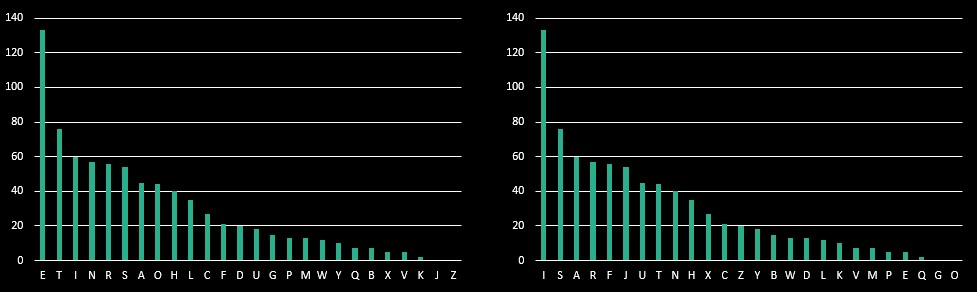###### Even more usefulness

We have seen that an index of coincidence of around 0.0660 can tell us that a Caesar, substitution or transposition cipher has probably been used, but what if the value of the index is lower than 0.0660 but higher than 0.0385, the index for random text?

In such cases, the index suggests that one possibility is that the cipher text has been created by a polyalphabetic cipher, like the Vigenère cipher. If the cipher had a long key and thus used lots of alphabets, then the closer the index will be to the index for random text, i.e. 0.0385. An index of coincidence of, say, 0.0550, might suggest a key of just 2 characters, whereas an index of around 0.0400 might come from a key length of around 8 characters. This is fine for a first initial impression when looking at the index of coincidence, but the index of coincidence offers us more precision when trying to ascertain the key length.

Let's look at the message from earlier in the page, and imagine that it has been enciphered with a key of 5 characters: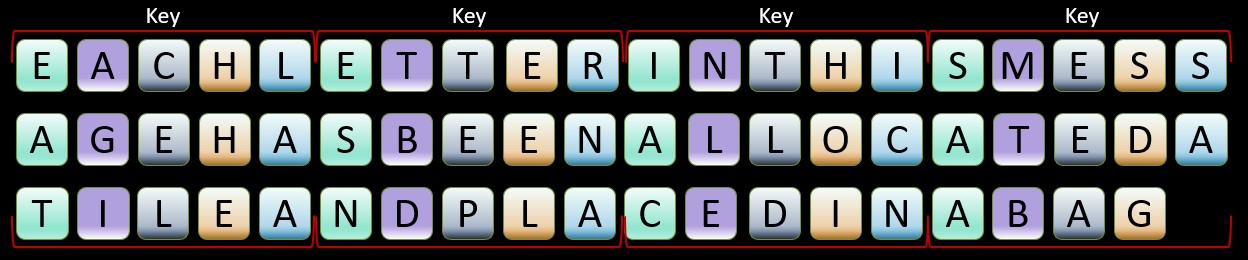We can extract each letter encoded by the same part of the key, i.e. all those that share the same colour in the image, and place them together in their own group. Each letter in a group has been encoded using the same Caesar cipher and this is because each letter has been encoded by the same part of the key.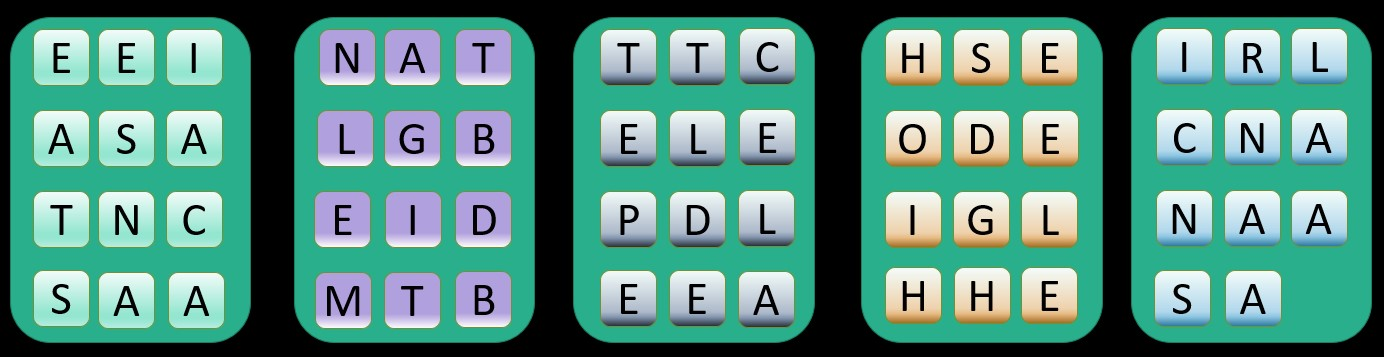Each group, therefore, contains a colleciton of letters that have been encoded using the same Caesar cipher. We should expect, therefore, the index of coincidence for just the letters in one individual group to be similar to the index of coincidence for normal English, i.e. around 0.0660. We can repeat this process for every group, and then find an average of all of the indexes of coincidence from the different groups. So: overall index of coincidence = sum of indexes of coincidences for each group divided by the number of groups. If we have got the right key length, then the average of all the individual indexes of coincidence should also work out at around 0.0660.

But what happens if we are working with the wrong key length? We can see what happens by looking again at the message from earlier. We are again imagining that it has been encoded by a key of 5 characters, but we are going to see what happens if we treat it as having a key length of 4 characters: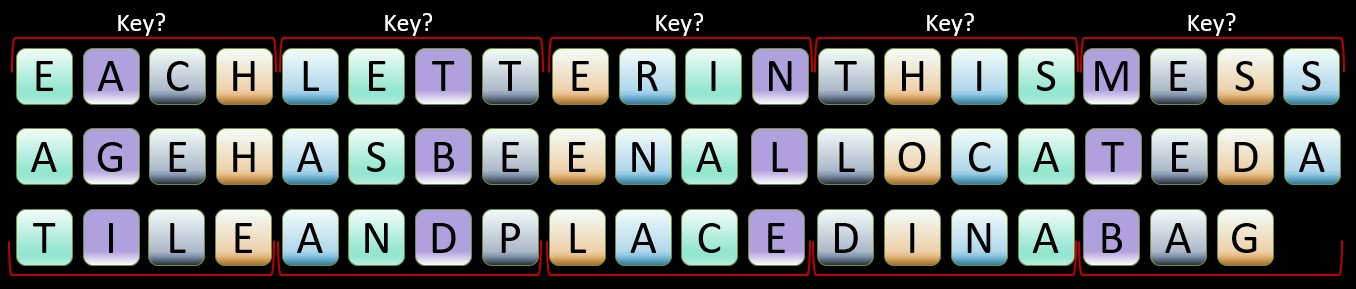If we now pull all the characters that have been encoded by the same part of the key into their own groups then we get the following: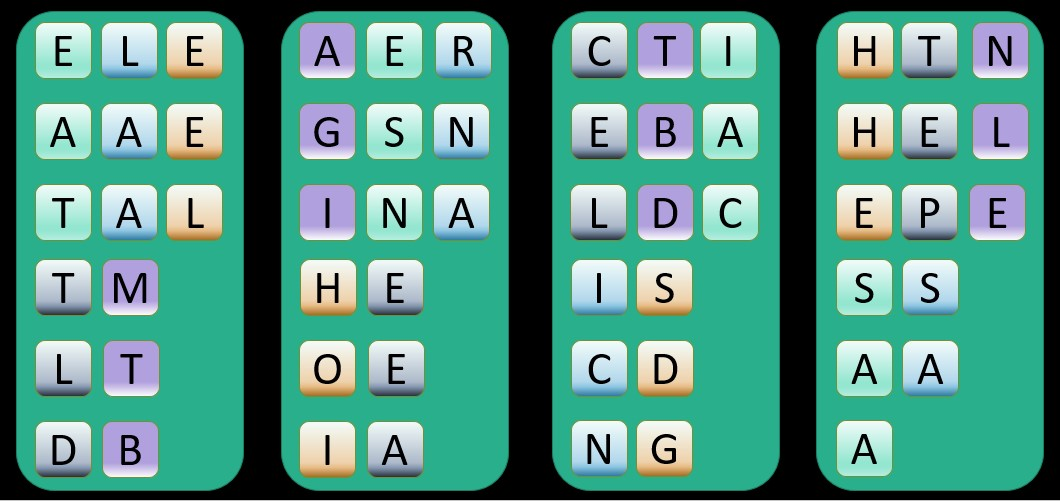Because we have got the key length wrong, we have ended up with groups of letters that have been encoded by different parts of the key or by different Caesar ciphers. If we now work out the index of coincidence for each group, then we will not get an index matching everday English.

If, therefore, we have a cipher text in front of us, we can get a computer to create the groups of letters based on different possible key lengths and then get it to work out the average index of coincidence for the groups for each key length. When the computer uses the correct key length, then the average index of coincidence should be higher. Let's see if this works:

XKIIMFUZZWPAJGWIUMBVNBEAJRRWIAXZKYBCRXMIGALXVCIXTHJAGLTKIFVNXIPYYPLIVEGJOCIDOBSWLHPPGILBTBJRKXKIGGILCSLQEUEFXSTNXHPBQMIXRJORVIXTMJRGFHGCOKPPCXJRGWRSBQTZTLNAIDGKXVGKKSDOCLHOHCKGYFBEUHKRFDGYVUQWTBCEUXRJWVGDPXWBSWLHWWZZERWJAEFXHVONYLWPBJWKMIXCHGMEARNHWSLXWPZEXHLJWHXKIZGTDIWXOXKINIMKYPDSROJHVHRHEGBHPALMSLHVGCXBTBYUSBIGFIVZETZNHPHRJXVEGKQTJWCV MCIOPJQWXBRWZLDXACQBHIQNZLKHRFGIFEWNAWRMQXSTKPIXWPMICRYOTKQGNRWKWSFVOEQFINXDVIMUWHRXLQANVMVXUCWPMQDLXAORYWLCYRLCVCQINIBMGDAQLTFRPOHHEZYOQWIQJXOEWIFHUOIWNVSPIQXBQZFRTQXALR WLSGDXBEUNEESYIHJKOTPANLVMQXVGIFEWNAXHBWXVGLFGHCQVHTUIGGTQHARWXKISPOKTQTMCCLHWHGCPJXCSOXYUXKVSRXBHTWCWDRGXVGZEXGMAISVHWSPZPTXOCLLWZIFGGJDCXJPSLDFSVOZRXYQIUAHACWRACDCRGHXK EHJGMETJAWPSVXCHZBCXWCLHGLTVGXQTMCJRGXKYGGDMTRCRXWSUIDTKPTCCNMQXKIDNGFCINGXEYWWIENXCPBBYPTWMCPCLJAMXROCEIFKMEIXWXRHSIXVGLLJGLJWHWKIFGGKSTENRWLDXWURXGVNUCECFSWPIFSTWLI

This is the text that we examined on the previous page and, by counting the gaps between repeating sequences of text, we guessed that the key length is 13. When we use the approach described above, we get the following average indexes of coincidence for these key lengths:

Key Length Index of Coincidence
1 0.04265
2 0.04251
3 0.04290
4 0.04206
5 0.04202
6 0.04277
7 0.04153
8 0.04213
9 0.04240
10 0.04251
11 0.04423
12 0.04256
13 0.07204
14 0.04008
15 0.04133

The average index of coincidence for a key length of 13 stands out, with a very high 0.07204. Although it is not shown here, the average index of coincidence for a key length of 26 is also very high: 0.07072. This is to be expected because 26 is a multiple of 13, the actual key length. If this situation arises, your instinct should be to check the shorter of the two key lengths first.

Both of our two methods suggest, therefore, that the key length is 13. It has taken some time to explain this second method based on the index of coincidence, but it has been worth it. It is a faster method than method 1, based on looking for repeated sequences of text, and it offers greater insight into the kind of cipher used to encrypt a message. Now that we have established the key length, we will look on the next page at how to use this to finally crack a Vigenère cipher.

This website automatically works out the index of coincidence for a message based on different key lengths for you. Click here and copy a cipher text in the top box. Then, select 'Frequency Analysis' from the drop-down options just below and click on the button. The index of coincidence for each key length will be displayed in the Output Text box, about halfway down.

Below you will find further mathematical details about the formula for the index of coincidece. These can be skipped.

#### Formula to calculate the Index of Coincidence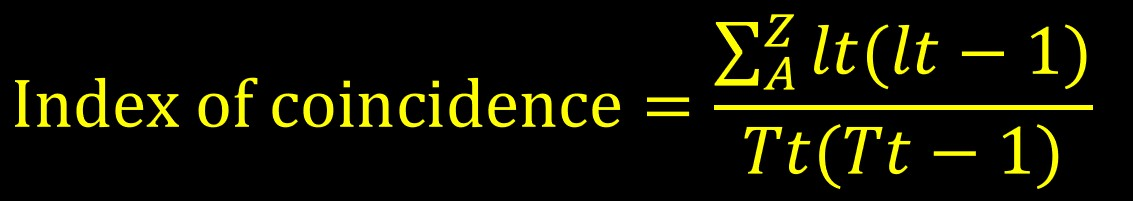Where lt is the number of times a particular letter occurs and Tt is the total number of letters in the message. The top half of the formula means that lt(lt-1) needs to be worked out separately for each letter between and including A to Z, and then these 26 totals need to be added together.

So the overall calculation looks like this: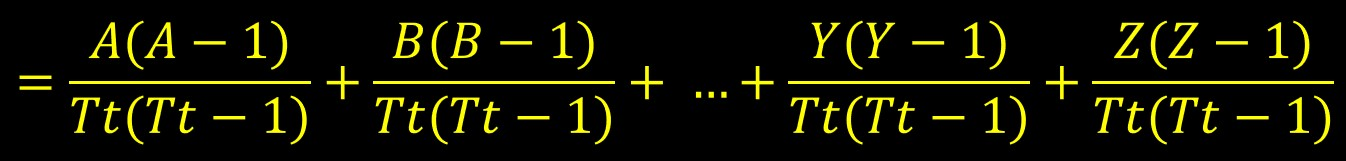To understand why this is the formula, we need to remember what the index of coincidence is calculating, namely the probabilitiy of extracting two identical letters from a message if all the letters have been placed on tiles and put in a bag. It is like the classic Maths question of the probability of drawing two same-coloured socks from a drawer of socks. The chance of drawing one 'A' tile is: the number of 'A' tiles divided by the total number of tiles. So the chance of drawing two 'A' tiles is as follows: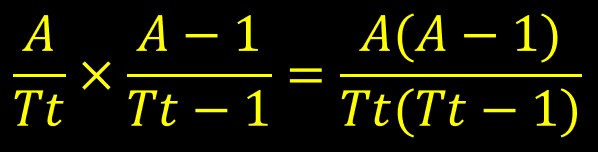The overall formula simply repeats this process for all of the 26 letters and adds all the results together.

#### The Index of Coincidence for random text

Random text is a section of text where each letter has an equal chance of occurring. The following is one such example:

ABCDEFGHIJKLMNOPQRSTUVWXYZABCDEFGHIJKLMNOPQRSTUVWXYZABCDEFGHIJKLMNOPQRSTUVWXYZABCDEFGHIJKLMNOPQRSTUVWXYZABCDEFGHIJKLMNOPQRSTUVWXYZABCDEFGHIJKLMNOPQRSTUVWXYZ

In this example, the whole of the alphabet has been repeated in full several times. If we use Tt to refer to the total number of letters in the text, then each letter occurs 26/Tt times. We can use this to rewrite the formula for the index of coincidence as follows: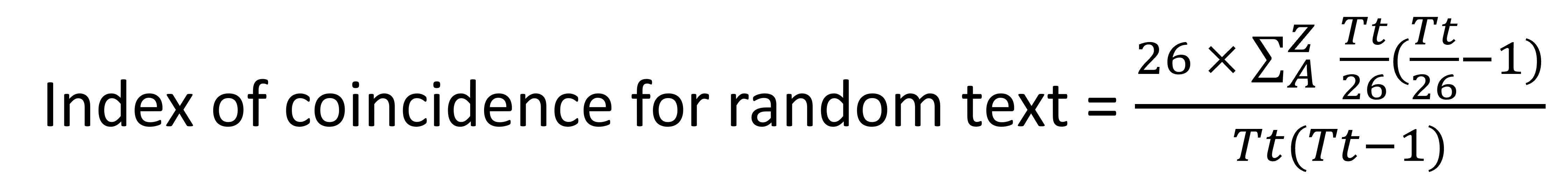We can simplify further and this is because, when we repeat the calculations for each of the 26 letters, we will get exactly the same answer, as they each occur the same amount of times: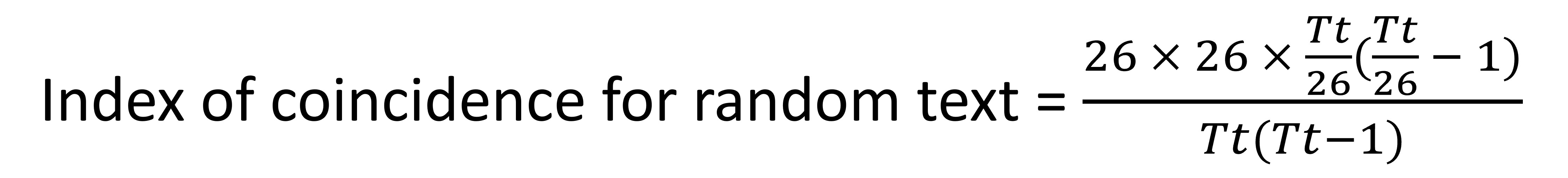With some further simplification: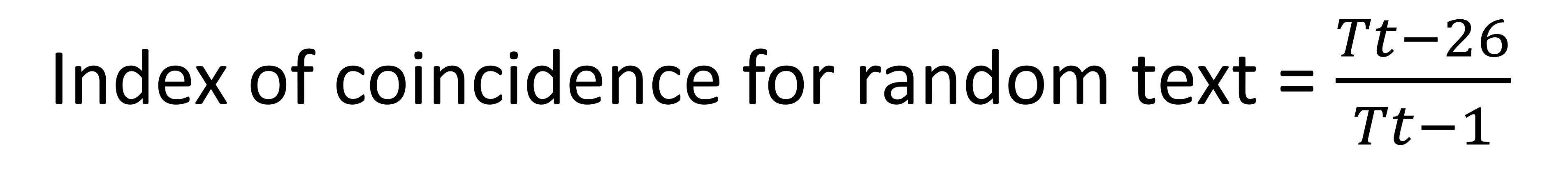We can plot this formula, using the 𝑥 axis for 𝑇𝑡, and the 𝑦 axis for the index of coincidence.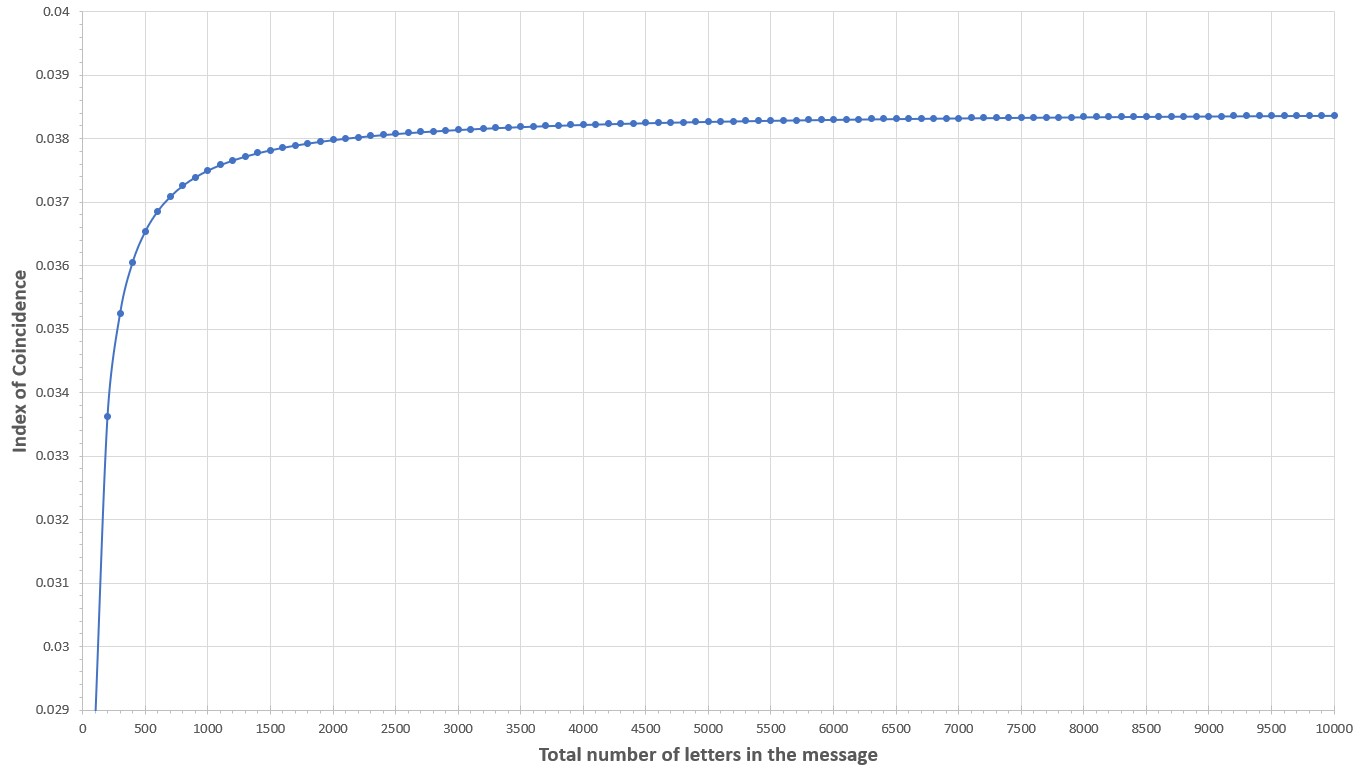From the graph we can see that, as Tt gets larger, so the graph gradually gets closer and closer to 0.0385. This is becasue, as Tt gets very large, the impact of the two '-26's in the formula becomes negligible, so the formula effectively becomes Tt divided by 26Tt, which is 1/26, or approximately 0.0385.

Cipher Challenge competition    Leave feedback